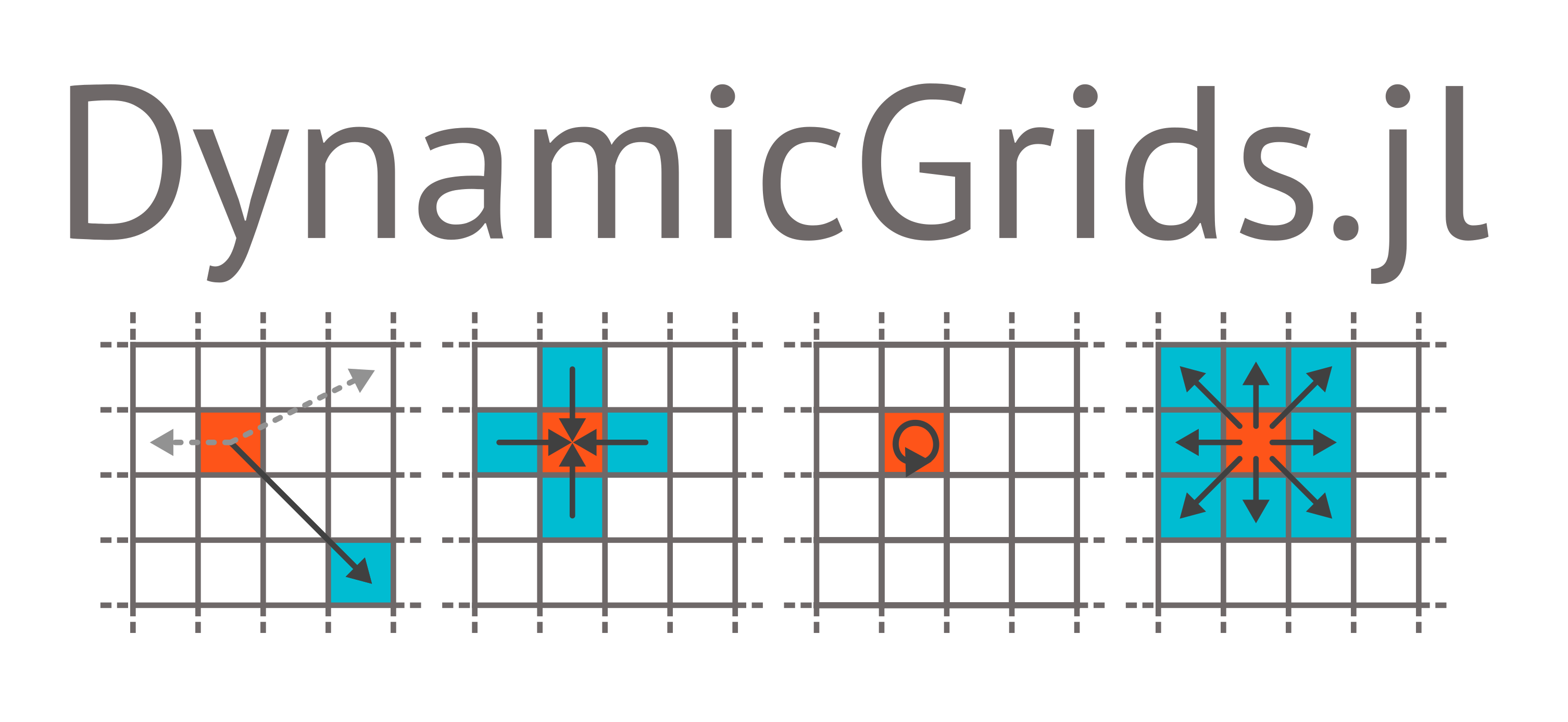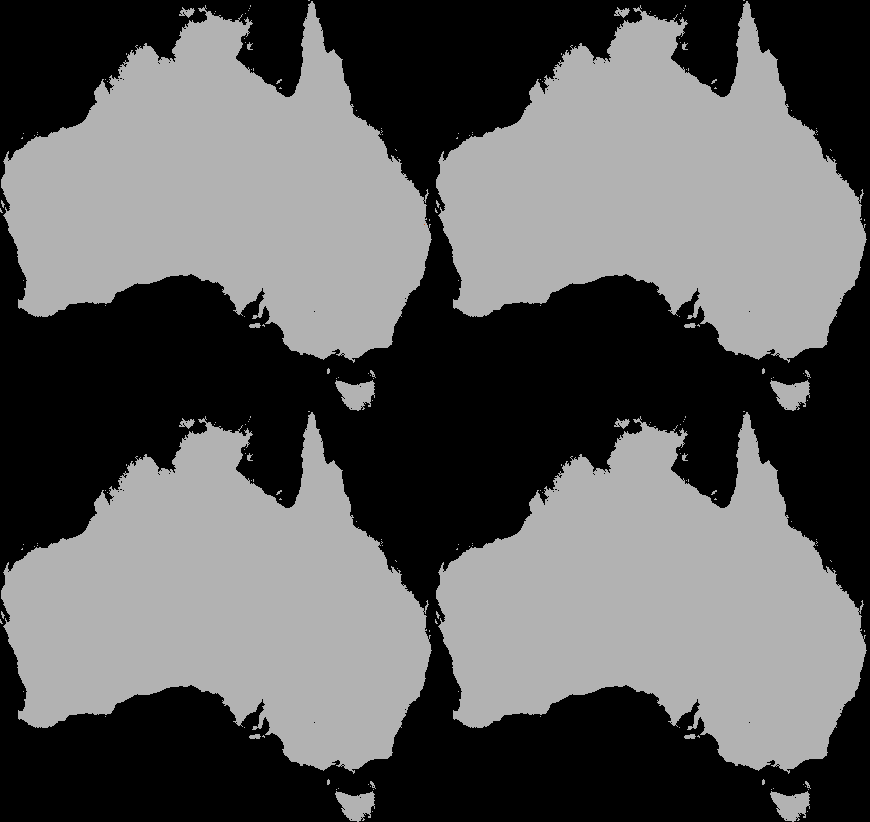## DynamicGrids.jl

Grid-based simulations in Julia
Author cesaraustralia
Popularity
112 Stars
Updated Last
2 Years Ago
Started In
June 2018DynamicGrids is a generalised framework for building high-performance grid-based spatial simulations, including cellular automata, but also allowing a wider range of behaviours like random jumps and interactions between multiple grids. It is extended by Dispersal.jl for modelling organism dispersal processes.

DynamicGridsGtk.jl provides a simple live interface, while DynamicGridsInteract.jl also has live control over model parameters while the simulation runs: real-time visual feedback for manual parametrisation and model exploration.

DynamicGrids can run rules on single CPUs, threaded CPUs, and on CUDA GPUs. Simulation run-time is usually measured in fractions of a second.A dispersal simulation with quarantine interactions, using Dispersal.jl, custom rules and the GtkOuput from DynamicGridsGtk. Note that this is indicative of the real-time frame-rate on a laptop.

A DynamicGrids.jl simulation is run with a script like this one running the included game of life model `Life()`:

```using DynamicGrids, Crayons

init = rand(Bool, 150, 200)
output = REPLOutput(init; tspan=1:200, fps=30, color=Crayon(foreground=:red, background=:black, bold=true))
sim!(output, Life())

# Or define it from scratch (yes this is actually the whole implementation!)
const sum_states = (false, false, true, false, false, false, false, false, false),
(false, false, true, true,  false, false, false, false, false)
life = Neighbors(Moore(1)) do hood, state
sum_states[state + 1][sum(hood) + 1]
end
sim!(output, life)```A game of life simulation being displayed directly in a terminal.

# Concepts

The framework is highly customisable, but there are some central ideas that define how a simulation works: grids, rules, and outputs.

## Grids

Simulations run over one or many grids, derived from `init` of a single `AbstractArray` or a `NamedTuple` of multiple `AbstractArray`. Grids (`GridData` types) are, however not a single array but both source and destination arrays, to maintain independence between cell reads and writes where required. These may be padded or otherwise altered for specific performance optimisations. However, broadcasted `getindex` operations are guaranteed to work on them as if the grid is a regular array. This may be useful running simulations manually with `step!`.

Usually grids contain values of `Number`, but other types are possible, such as `SArray`, `FieldVector` or other custom structs. Grids are updated by `Rule`s that are run for every cell, at every timestep.

The `init` grid/s contain whatever initialisation data is required to start a simulation: the array type, size and element type, as well as providing the initial conditions:

``````init = rand(Float32, 100, 100)
``````

An `init` grid can be attached to an `Output`:

``````output = ArrayOutput(init; tspan=1:100)
``````

or passed in to `sim!`, where it will take preference over the `init` attached to the `Output`, but must be the same type and size:

``````sim!(output, ruleset; init=init)
``````

For multiple grids, `init` is a `NamedTuple` of equal-sized arrays matching the names used in each `Ruleset` :

`init = (predator=rand(100, 100), prey=(rand(100, 100))`

Handling and passing of the correct grids to a `Rule` is automated by DynamicGrids.jl, as a no-cost abstraction. `Rule`s specify which grids they require in what order using the first two (`R` and `W`) type parameters.

Dimensional or spatial `init` grids from DimensionalData.jl or GeoData.jl will propagate through the model to return output with explicit dimensions. This will plot correctly as a map using Plots.jl, to which shape files and observation points can be easily added.

### Non-Number Grids

Grids containing custom and non-`Number` types are possible, with some caveats. They must define `Base.zero` for their element type, and should be a bitstype for performance. Tuple does not define `zero`. `Array` is not a bitstype, and does not define `zero`. `SArray` from StaticArrays.jl is both, and can be used as the contents of a grid. Custom structs that defne `zero` should also work.

However, for any multi-values grid element type, you will need to define a method of `DynamicGrids.to_rgb` that returns an `ARGB32` for them to work in `ImageOutput`s, and `isless` for the `REPLoutput` to work. A definition for multiplication by a scalar `Real` and addition are required to use `Convolution` kernels.

## Rules

Rules hold the parameters for running a simulation, and are applied in `applyrule` method that is called for each of the active cells in the grid. Rules come in a number of flavours (outlined in the docs), which allow assumptions to be made about running them that can greatly improve performance. Rules can be collected in a `Ruleset`, with some additional arguments to control the simulation:

``````ruleset = Ruleset(Life(2, 3); opt=SparseOpt(), proc=CuGPU())
``````

Multiple rules can be combined in a `Ruleset` or simply passed to `sim!`. Each rule will be run for the whole grid, in sequence, using appropriate optimisations depending on the parent types of each rule:

`ruleset = Ruleset(rule1, rule2; timestep=Day(1), opt=SparseOpt(), proc=ThreadedCPU())`

## Output

Outputs are ways of storing or viewing a simulation. They can be used interchangeably depending on your needs: `ArrayOutput` is a simple storage structure for high performance-simulations. As with most outputs, it is initialised with the `init` array, but in this case it also requires the number of simulation frames to preallocate before the simulation runs.

`output = ArrayOutput(init; tspan=1:10)`

The `REPLOutput` shown above is a `GraphicOutput` that can be useful for checking a simulation when working in a terminal or over ssh:

`output = REPLOutput(init; tspan=1:100)`

`ImageOutput` is the most complex class of outputs, allowing full color visual simulations using ColorSchemes.jl. It can also display multiple grids using color composites or layouts, as shown above in the quarantine simulation.

DynamicGridsInteract.jl provides simulation interfaces for use in Juno, Jupyter, web pages or electron apps, with live interactive control over parameters, using ModelParameters.jl. DynamicGridsGtk.jl is a simple graphical output for Gtk. These packages are kept separate to avoid dependencies when being used in non-graphical simulations.

Outputs are also easy to write, and high performance applications may benefit from writing a custom output to reduce memory use, or using `TransformedOuput`. Performance of DynamicGrids.jl is dominated by cache interactions, so reducing memory use has positive effects.

## Example

This example implements the classic stochastic forest fire model in a few different ways, and benchmarks them.

First we will define a Forest Fire algorithm that sets the current cell to burning, if a neighbor is burning. Dead cells can come back to life, and living cells can spontaneously catch fire:

```using DynamicGrids, ColorSchemes, Colors, BenchmarkTools

const DEAD, ALIVE, BURNING = 1, 2, 3

neighbors_rule = let prob_combustion=0.0001, prob_regrowth=0.01
Neighbors(Moore(1)) do neighborhood, cell
if cell == ALIVE
if BURNING in neighborhood
BURNING
else
rand() <= prob_combustion ? BURNING : ALIVE
end
elseif cell == BURNING
else
rand() <= prob_regrowth ? ALIVE : DEAD
end
end
end

# Set up the init array and output (using a Gtk window)
init = fill(ALIVE, 400, 400)
output = GifOutput(init;
filename="forestfire.gif", tspan=1:200, fps=25,
imagegen=Image(scheme=ColorSchemes.rainbow, zerocolor=RGB24(0.0))
)

# Run the simulation, which will save a gif when it completes
sim!(output, neighbors_rule)```Timing the simulation for 200 steps, the performance is quite good. This particular CPU has six cores, and we get a 5.25x speedup by using all of them, which indicates good scaling:

```bench_output = ResultOutput(init; tspan=1:200)

julia> @btime sim!(\$bench_output, \$neighbors_rule);
477.183 ms (903 allocations: 2.57 MiB)

91.321 ms (15188 allocations: 4.07 MiB)```

We can also invert the algorithm, setting cells in the neighborhood to burning if the current cell is burning, by using the `SetNeighbors` rule:

```setneighbors_rule = let prob_combustion=0.0001, prob_regrowth=0.01
SetNeighbors(Moore(1)) do data, neighborhood, cell, I
if rand() <= prob_regrowth
data[I...] = ALIVE
end
elseif cell == BURNING
for pos in positions(neighborhood, I)
if data[pos...] == ALIVE
data[pos...] = BURNING
end
end
elseif cell == ALIVE
if rand() <= prob_combustion
data[I...] = BURNING
end
end
end
end```

Note: we are not using `add!`, instead we just set the grid value directly. This usually risks errors if multiple cells set different values. Here they only ever set a currently living cell to burning in the next timestep. It doesn't matter if this happens multiple times, the result is the same.

And in this case (a fairly sparse simulation), this rule is faster:

```julia> @btime sim!(\$bench_output, \$setneighbors_rule);
261.969 ms (903 allocations: 2.57 MiB)

65.489 ms (7154 allocations: 3.17 MiB)```

But the scaling is not quite as good, at 3.9x for 6 cores. The first method may be better on a machine with a lot of cores.

Last, we can slightly rewrite these rules for GPU, as `rand` is not available within a GPU kernel. Instead we call `CUDA.rand!` on the entire parent array of the `:rand` grid, using a `SetGrid` rule:

```using CUDAKernels, CUDA

randomiser = SetGrid{Tuple{},:rand}() do randgrid
CUDA.rand!(parent(randgrid))
end```

Now we define a Neighbors version for GPU, using the `:rand` grid values instead of `rand()`:

```neighbors_gpu = let prob_combustion=0.0001, prob_regrowth=0.01
Neighbors{Tuple{:ff,:rand},:ff}(Moore(1)) do neighborhood, (cell, rand)
if cell == ALIVE
if BURNING in neighborhood
BURNING
else
rand <= prob_combustion ? BURNING : ALIVE
end
elseif cell == BURNING
else
rand <= prob_regrowth ? ALIVE : DEAD
end
end
end```

And a SetNeighbors version for GPU:

```setneighbors_gpu = let prob_combustion=0.0001, prob_regrowth=0.01
SetNeighbors{Tuple{:ff,:rand},:ff}(Moore(1)) do data, neighborhood, (cell, rand), I
if rand <= prob_regrowth
data[:ff][I...] = ALIVE
end
elseif cell == BURNING
for pos in positions(neighborhood, I)
if data[:ff][pos...] == ALIVE
data[:ff][pos...] = BURNING
end
end
elseif cell == ALIVE
if rand <= prob_combustion
data[:ff][I...] = BURNING
end
end
end
end```

Now we benchmark both version on a GTX 1080. Despite the overhead of reading and writing two grids, this turns out to be even faster again:

```bench_output_rand = ResultOutput((ff=init, rand=zeros(size(init))); tspan=1:200)

julia> @btime sim!(\$bench_output_rand, \$randomiser, \$neighbors_gpu; proc=CuGPU());
30.621 ms (186284 allocations: 17.19 MiB)

julia> @btime sim!(\$bench_output_rand, \$randomiser, \$setneighbors_gpu; proc=CuGPU());
22.685 ms (147339 allocations: 15.61 MiB)```

That is, we are running the rule at a rate of 1.4 billion times per second. These timings could be improved (maybe 10-20%) by using grids of `Int32` or `Int16` to use less memory and cache. But we will stop here!

## Alternatives

Agents.jl can also do cellular-automata style simulations. The design of Agents.jl is to iterate over a list of agents, instead of broadcasting over an array of cells. This approach is well suited to when you need to track the movement and details about individual agents throughout the simulation.

However, for simple grid models where you don't need to track individuals, DynamicGrids.jl is orders of magnitude faster than Agents.jl, and usually requires less code to define a model. For low-density simulations like the forest fire model above, it can be one or two orders of magnitudes faster, while for higher activity rules like the game of life on a randomised grid, it is two to three, even four order of magnitude faster, increasing with grid size. If you are doing grid-based simulation and you don't need to track individual agents, DynamicGrids.jl is probably the best tool. For other use cases where you need to track individuals, try Agents.jl.